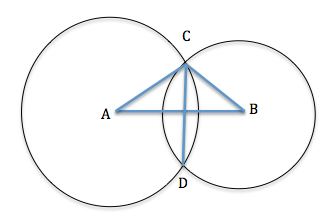### Two circles of radii 13 cm and 5 cm intersect at two points and the distance between their centres is 12 cm. Find the length of the common chord.

10 cm

Step by Step Explanation:
1. Take a look at the following image:Here, A is the center of the first circle and B the center of the second circle. The common chord is CD.
Join AD and BD. Now, consider ∠ABC and ∠ABD. We have,
BC = BD (Radius of the circle with centre B)
AB = AB (common)
Hence, ∠ABC ≅ ∠ABD by SSS.
So, ∠AOC = ∠AOD by CPCT. Also, AO = OB and CO = DO by CPCT.
Also, ∠AOC + ∠AOD = 180° (angles on a straight line)
⇒ ∠AOC + ∠AOC = 180°
⇒ 2 × ∠AOC = 180°
⇒ ∠AOC = 90°

Now, we know that the line AB bisects CD, and is perpendicular to it.
Also, the perpendicular from C to AB is half the length of CD. Let us call this length as L.
Area of ΔABC =
 1 2
× AB × L
2. By Heron's formula, area of ΔABC = $\sqrt{ S(S-a)(S-b)(S-c) }$,
where, S =
 AB + BC + CA 2
=
 13 + 5 + 12 2
= 15 cm
and a,b, and c are the length of three sides of the triangle. So, area of ΔABC = $\sqrt{ 15(15-13)(15-5)(15-12) }$ = 30 cm
3. Area of ΔABC = 30 cm =
 1 2
× AB × L =
 1 2
× 12 × L
Therefore, L =
 2 × 30 12
= 5 cm
Length of CD = 2L = 2 × 5 cm = 10 cm